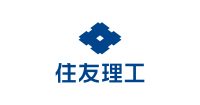# Sumitomo Riko Co Ltd (TSE:5191)

555 JPY +5 JPY ( +0.91% )Sumitomo Riko Co Ltd
TSE:5191

-

-

## FAQ

### What is Discount Rate?

Discount rate reflects the opportunity cost of investment (i.e. the return that could be earned on investment with similar risk).

### Cost of Equity Calculation

Cost of Equity
-
Rf
-
Beta
-
ERP
-

The Cost of Equity for Sumitomo Riko Co Ltd (TSE:5191) calculated via CAPM (Capital Asset Pricing Model) is -.

### WACC Calculation

WACC
-

Cost of Equity
-
Equity Weight
-

Cost of Debt
-
Debt Weight
-

The WACC for Sumitomo Riko Co Ltd (TSE:5191) is -.